# How to Calculate and Solve for Total Cross-Sectional Area of Gates | Design of Gating System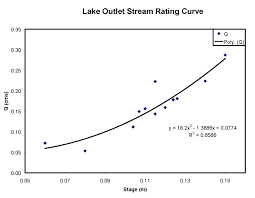The image above represents total cross-sectional area of gates.

To compute for total cross-sectional area of gates, three essential parameters are needed and these parameters are Mass of the Casting (G), Specific Speed of Pouring (Ks) and Time of Pouring (t).

The formula for calculating total cross-sectional area of gates:

Fs = G / tKs

Where:

Fs = Total Cross-Sectional Area of Gates
G = Mass of the Casting
Ks = Specific Speed Of Pouring
t = Time of Pouring

Let’s solve an example;
Find the total cross-sectional area of gates when the mass of the casting is 10, specific speed of pouring is 18 and the time of pouring is 9.

This implies that;

G = Mass of the Casting = 10
Ks = Specific Speed Of Pouring = 18
t = Time of Pouring = 9

Fs = G / tKs
Fs = 10 / 9 x 18
Fs = 10 / 162
Fs = 0.0617

Therefore, the total cross-sectional area of gates is 0.0617 m2.

Calculating the Mass of the Casting when the Total Cross-Sectional Area of Gates, Specific Speed of Pouring and the Time of Pouring is Given.

G = Fs x tKs

Where:

G = Mass of the Casting
Fs = Total Cross-Sectional Area of Gates
Ks = Specific Speed Of Pouring
t = Time of Pouring

Let’s solve an example;
Find the mass of the casting when the total cross-sectional area of gates is 15, the specific speed of pouring is 7 and the time of pouring is 5.

This implies that;

Fs = Total Cross-Sectional Area of Gates = 15
Ks = Specific Speed Of Pouring = 7
t = Time of Pouring = 5

G = Fs x tKs
G = 15 x 5(7)
G = 15 x 35
G = 525

Therefore, the mass of the casting is 525 m.

Calculating the Specific Speed of Pouring when the Total Cross-Sectional Area of Gates, Mass of the Casting and the Time of Pouring is Given.

Ks = G / Fs x t

Where;

Ks = Specific Speed Of Pouring
Fs = Total Cross-Sectional Area of Gates
G = Mass of the Casting
t = Time of Pouring

Let’s solve an example;
Find the specific speed of pouring when the total cross-sectional area of gates is 20, the mass of the casting is 18 and the time of pouring is 10.

This implies that;

Fs = Total Cross-Sectional Area of Gates = 20
G = Mass of the Casting = 18
t = Time of Pouring = 10

Ks = G / Fs x t
Ks = 18 / 20 x 10
Ks = 18 / 200
Ks = 0.09

Therefore, the specific speed of pouring is 0.09.

Calculating the Time of Pouring when the Total Cross-Sectional Area of Gates, the Mass of the Casting and the Specific Speed of Pouring is Given.

t = G / Fs x Ks

Where;

t = Time of Pouring
Fs = Total Cross-Sectional Area of Gates
G = Mass of the Casting
Ks = Specific Speed Of Pouring

Let’s solve an example;
Find the time of pouring when the total cross-sectional area of gates is 32, the mass of the casting is 22 and the specific speed of pouring is 14.

This implies that;

Fs = Total Cross-Sectional Area of Gates = 32
G = Mass of the Casting = 22
Ks = Specific Speed Of Pouring = 14

t = G / Fs x Ks
t = 22 / 32 x 14
t = 22 / 448
t = 0.049

Therefore, the time of pouring is 0.049.

Nickzom Calculator – The Calculator Encyclopedia is capable of calculating the total cross-sectional area of gates.

To get the answer and workings of the total cross-sectional area of gates using the Nickzom Calculator – The Calculator Encyclopedia. First, you need to obtain the app.

You can get this app via any of these means:

To get access to the professional version via web, you need to register and subscribe for NGN 1,500 per annum to have utter access to all functionalities.
You can also try the demo version via https://www.nickzom.org/calculator

Apple (Paid) – https://itunes.apple.com/us/app/nickzom-calculator/id1331162702?mt=8
Once, you have obtained the calculator encyclopedia app, proceed to the Calculator Map, then click on Materials & Metallurgical under Engineering.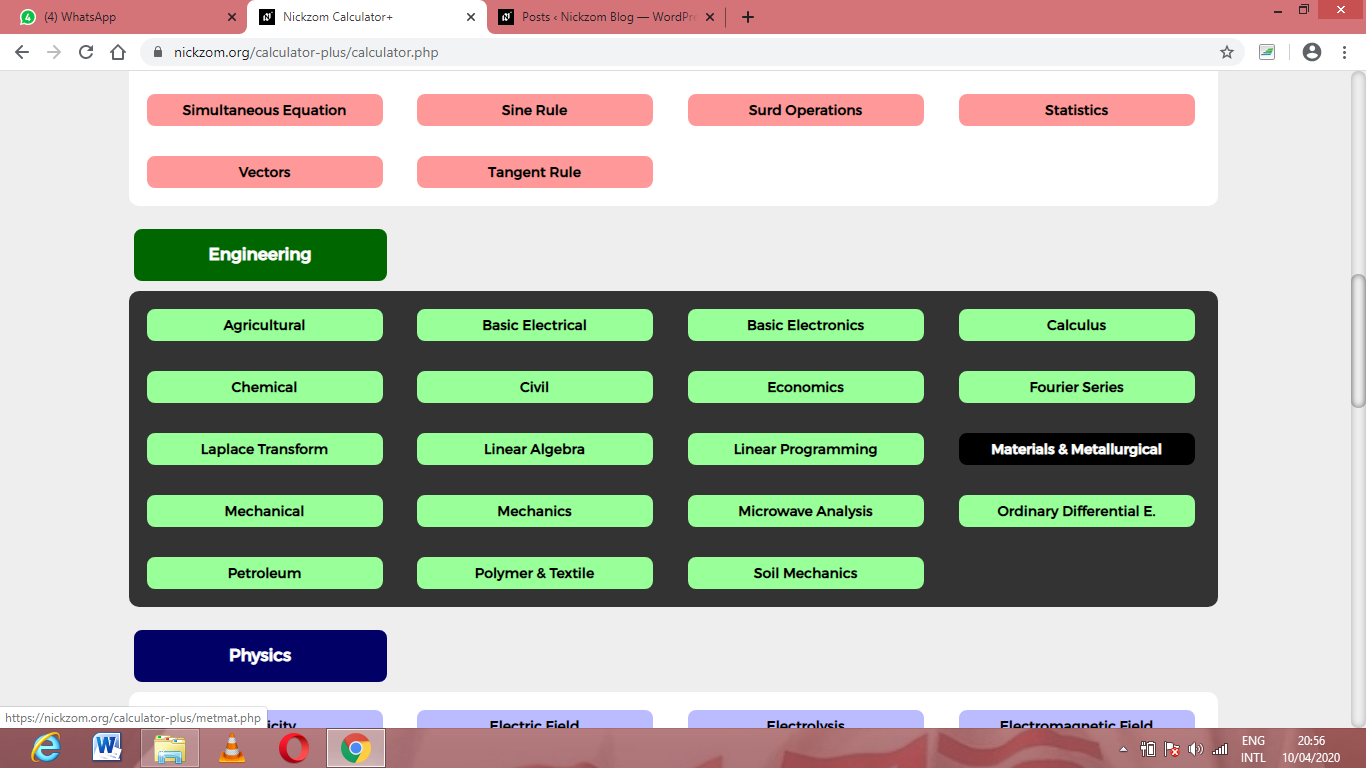Now, Click on Foundry Technology under Material & MetallurgicalNow, Click on Design of Gating System under Foundry Technology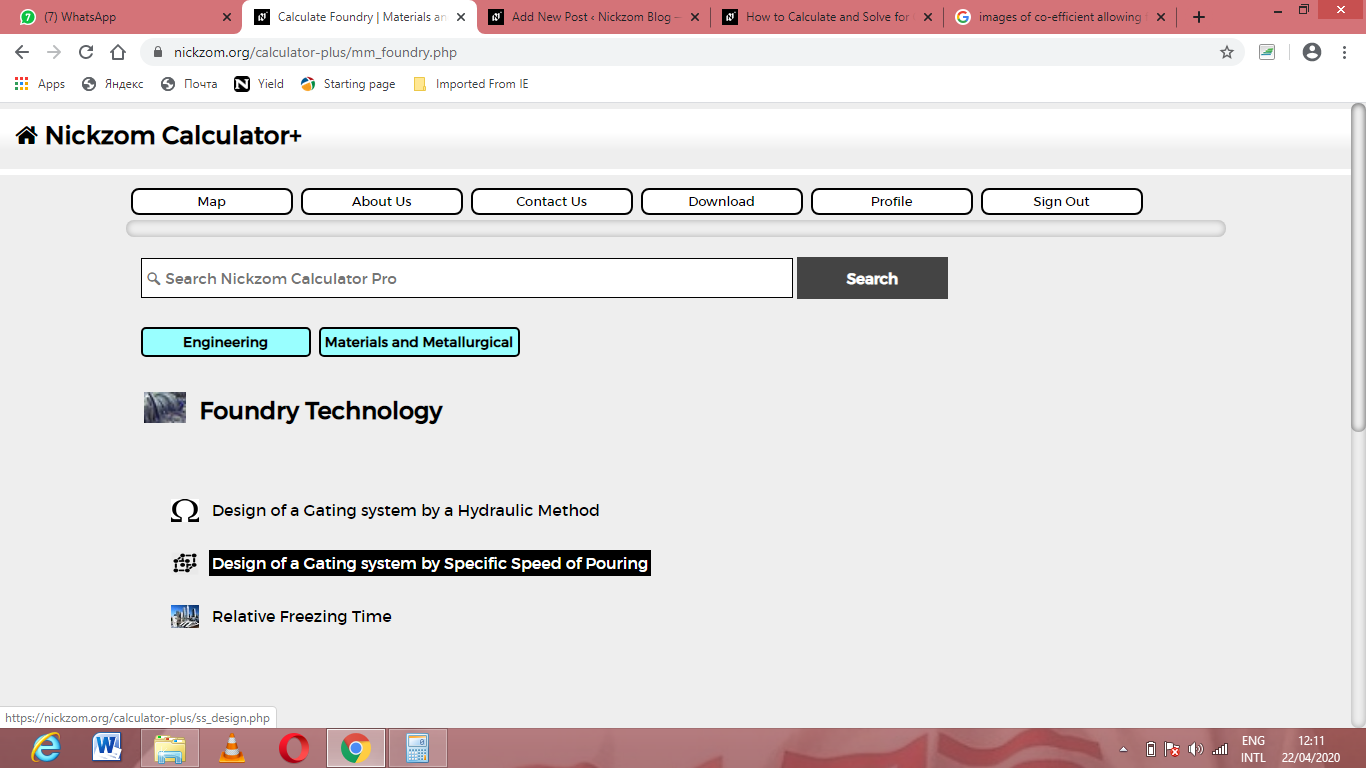Now, Click on Total Cross-Sectional Area of Gates under Design of Gating System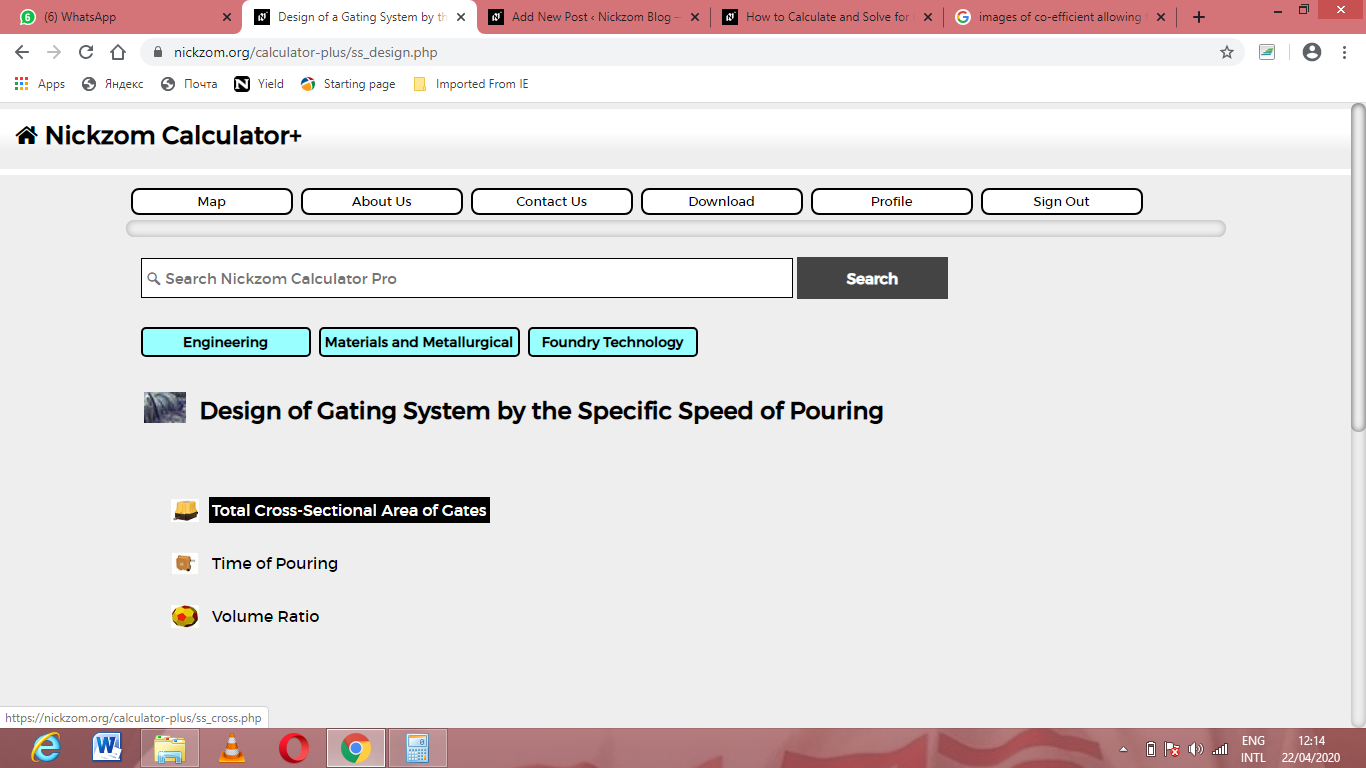The screenshot below displays the page or activity to enter your values, to get the answer for the total cross-sectional area of gates according to the respective parameters which are the Mass of the Casting (G), Specific Speed of Pouring (Ks) and Time of Pouring (t).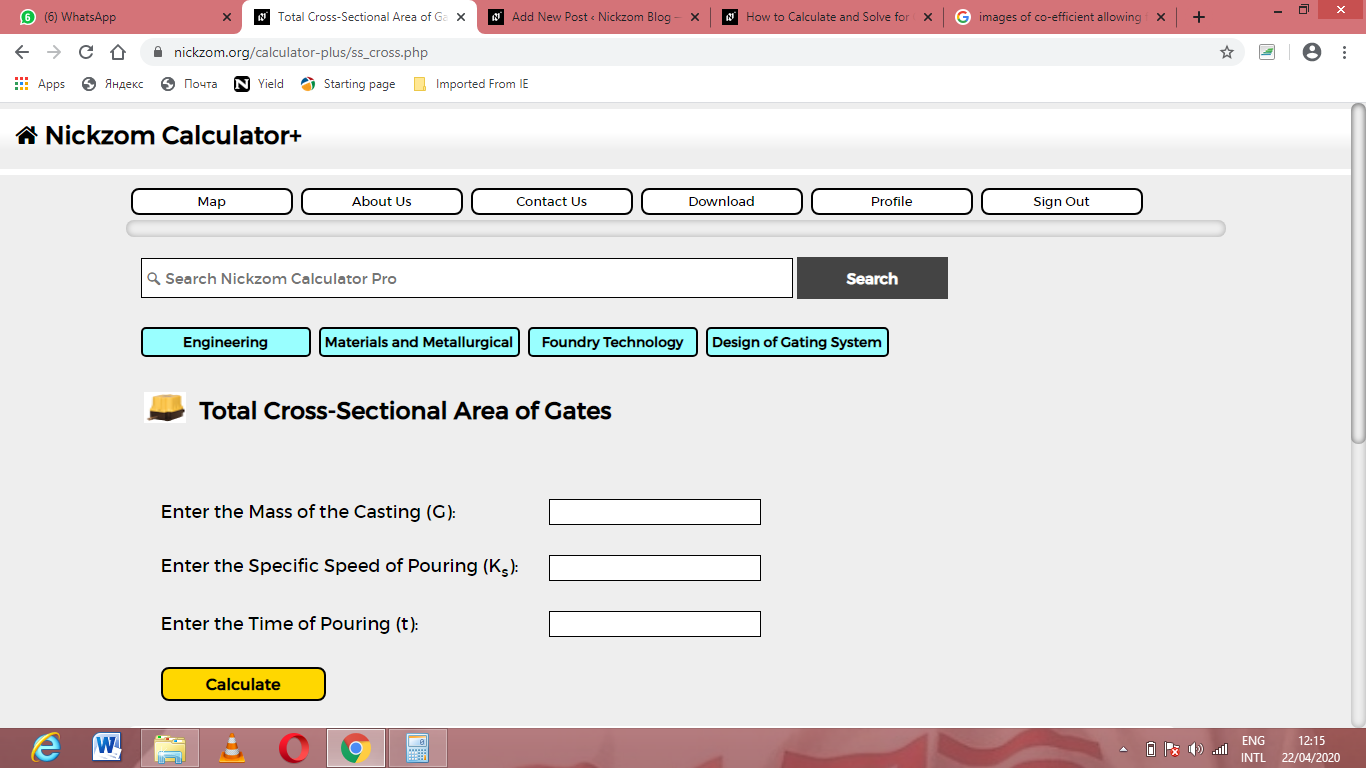Now, enter the values appropriately and accordingly for the parameters as required by the Mass of the Casting (G) is 10, Specific Speed of Pouring (Ksis 18 and Time of Pouring (t) is 9.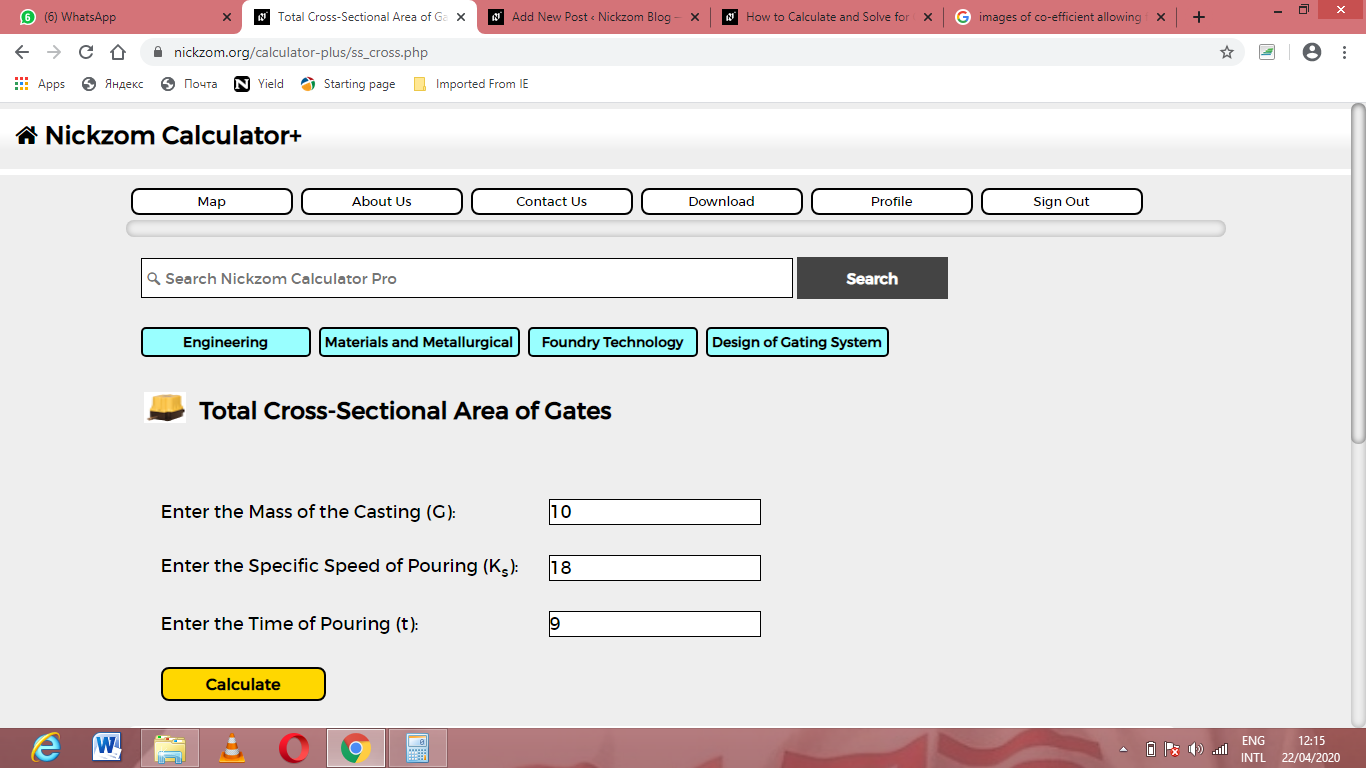Finally, Click on Calculate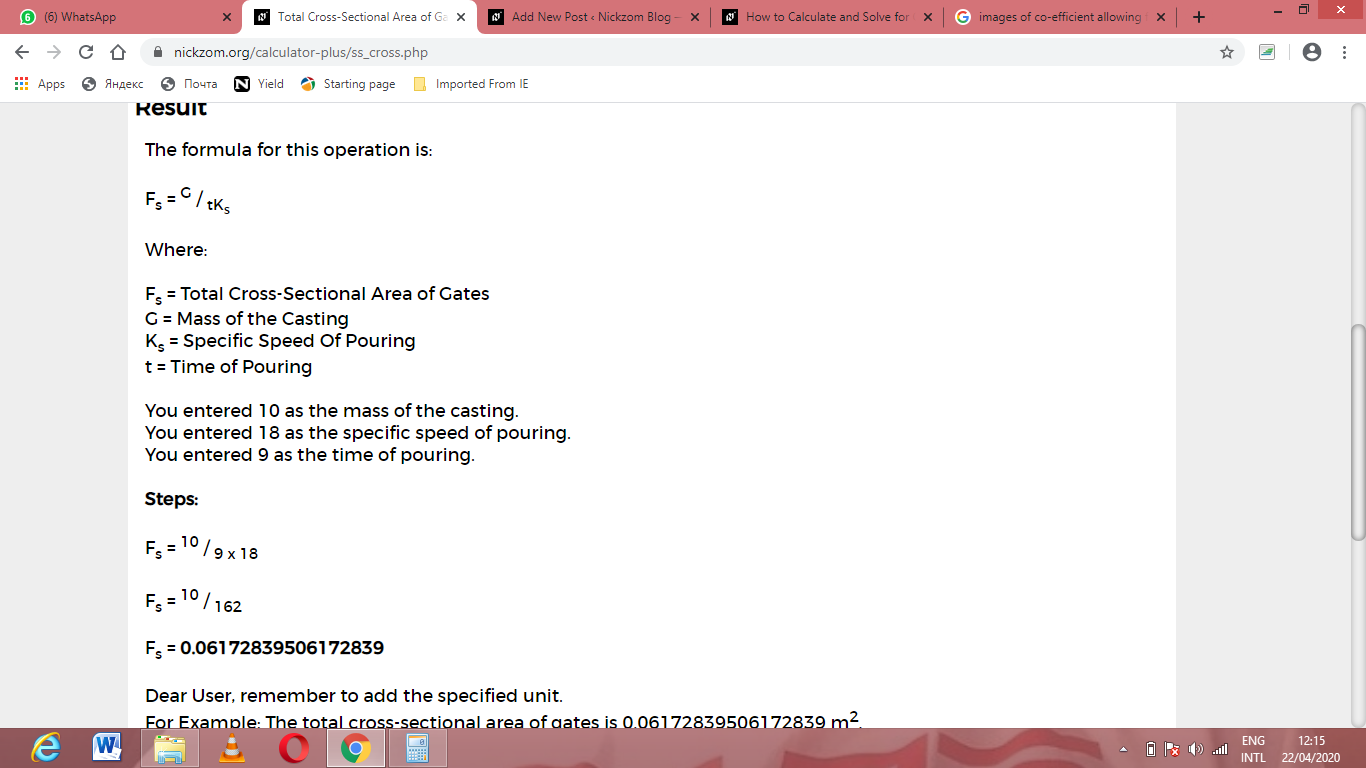As you can see from the screenshot above, Nickzom Calculator– The Calculator Encyclopedia solves for the total cross-sectional area of gates and presents the formula, workings and steps too.